Ecological Archives E094-258-A2

Emily V. Moran, Sharon Bewick, Christina A. Cobbold. 2013. Effects of plant genotype and insect dispersal rate on the population dynamics of a forest pest. Ecology 94:2792–2802. http://dx.doi.org/10.1890/12-1708.1

Appendix B. Further details on parameter estimation.

Alpha:

The value of αi depends on what life stage the caterpillar is in when the parasitoid emerges. A variety of host-specific parasitoids affect forest tent caterpillars, including Aleiodes malacosomatus (which emerges from 3rd or 4th instar), Leshenaultia exul (5th instar or pupa), Carcelia malacosomae (late 5th instar or pupa), Patelloa pachypyga (pupa) and Arachnidomyia aldrichi (late pupa) (Parry 1994). For this analysis, we do not specify a particular parasitoid. The values of αi shown in table 1 of the paper assume that parasitoid emergence time (PET), the day of caterpillar development on which the parasitoid emerges, is fixed at 37. The effects of varying PET can be seen in Fig. 2 of the paper and in the section entitled “Effect of PET” below.

We use aspen clone 271 as the reference clone, as it has the intermediate development time; average FTC development times ranged from 43 days on clone 259 to 48.4 days on clone 216. Whenever time to FTC pupation is lengthened or shortened relative to the reference clone, we assume that each FTC lifestage is lengthened or shortened proportionally. We partition the FTC development time on this clone into the average times spent in each caterpillar lifestage, denoted by the vector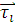. FTC go through 5 larval instars. We assume that the time spent in each of the first two instars is roughly half the time spent in each of the subsequent instars (Hwang and Lindroth 1997, Verdinelli and Sanna-Passino 2003). Density-dependent mortality occurs in the 4th and 5th instars, when caterpillars are large enough and have consumed enough of the initial leaf mass to compete strongly for food. The last few days of the 5th instar are alternately categorized as the “pre-pupal” stage.  After that time, the individual is a pupa, and no density-dependent mortality occurs. Thus, we assume that on clone 271: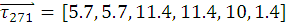(B.1)

where we have explicitly divided the 5th instar into an initial 10-day stage in which density-dependent mortality occurs and a 1.4-day “pre-pupal” stage.

We estimate strength of density-dependent competition for each FTC lifestage j, Kj,using data from Fig. 6 of Cobbold et al. (2009). The slopes of the lines in the figure provide estimates of r/Kj where r = 0.96 and Kjis measured in units of number per leaf cluster. Density-dependent mortality does not operate in the first three stages, so we set K1 = K2 = K3 =10,000, an arbitrary large constant, and the remaining values are found to be K4 =0.508, K5 =0.432 and K6 = 9.1485. Consequently, the overall strength of density-dependent competition over the entire season is given by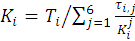(B.2)

where Ti is the total time to pupation on clone i, τi,jis the duration of stage j on clone i, giving K271= 1.5897 for the reference clone. The parameter Kjdoes not change between clones, but τi,j does. As the duration of each instar is scaled relative to the reference genome, it follows from Eq. (B.2) that K is the same for each clone. We therefore non-dimensionalize the model to scale host and parasitized-host density by this value of K. The non-dimensional version of the model presented in Eq. (2) has non-dimensional parameter K=1 for each clone.

The parameter αi is defined as follows for aspen clone i: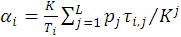(B.3)

where L is the FTC developmental stage at which the parasitoid emerges, and pj is the proportion of stage j density-dependent competition experienced by the parasitized host caterpillar. Hence, for a parasitoid with PET = 37 attacking hosts on the reference clone L=5, since instar 5 occurs between day 34.2 and day 44.2. Thus p4= 1, as the parasitoid is still in the host at the end of stage 4, and p5 = 0.28 because the parasitoid emerges from the host 28% the way through stage 5. This yields a271= 0.632.

Intrinsic rate of growth, r:

In order to calculate ri for each clone, we first used the mean values for female pupal mass and the linear relationship between pupal mass and egg number identified by Parry et al. (2001). Because there is a geographical cline in this relationship (southern populations produce a larger number of smaller eggs for a given female mass) we used the equation for Michigan, the population nearest the Wisconsin FACE site. For this Midwestern population: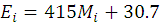(B.4)

where Ei is the number of eggs and Miis female pupal mass in grams. Next, because only around 2% of FTC eggs ultimately become adults (Sippell 1957), and because only female moths contribute to population growth, we convert from egg number to geometric growth rate using the relationship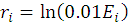(B.5)

Average female pupal masses ranged from 0.267 g on clone 216 to 0.316 g on clone 259. The resulting ri values are shown in Table 1 of the paper. These values are relatively small, because the pupal masses observed in the Aspen FACE experiments were at the low end of the potential weight range of 0.1 to 0.8 grams observed in wild populations (Parry et al. 2001).

Parameters independent of aspen clone:

Values for the remaining parameters, searching efficiency a and the degree of clumping of parasitoid searching k, were based on Cobbold et al. (2009) and references therein, giving k=100, close to random searchingand a ranging from 0.0085 to 0.03 in units of 1/(cocoons/area). However, because host and parasitoid density are expressed in number per leaf cluster in the data used to determine α and K we must first change the units of a. Cobbold et al. (2009) give K in terms of cocoons per area as 266.7 and we have K = 1.5897 in number per leaf cluster. These values should be equivalent; hence we can use this information to express a in units of 1/(number per leaf-cluster) by multiplying by 266.7/1.5897. In the non-dimensional model parasitoids are scale by K, hence the parameter a in Eq. (2) becomes non-dimensional via multiplication by K. We obtain the non-dimensional parameter a = 266.7(0.022) = 6.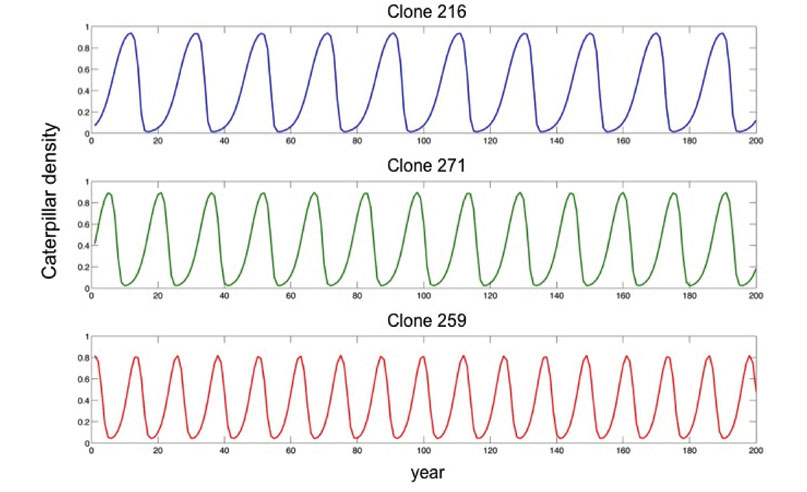Fig. B1. FTC population oscillations on isolated aspen clones, PET = 37.

Literature cited

Cobbold, C. A., J. Roland, and M. A. Lewis. 2009. The impact of parasitoid emergence time on host-parasitoid population dynamics. Theoretical Population Biology 75:201–215.

Hwang, S. Y., and R. L. Lindroth. 1997. Clonal variation in foliar chemistry of aspen: effects on gypsy moths and forest tent caterpillars. Oecologia 111:99–108.

Parry, D. 1994. The impact of predators and parasitoids on natural and experimentally created populations of forest tent caterpillar, Malacosoma

disstria Hubner (Lepidoptera: Lasiocampidae). University of Alberta, Edmonton, Alberta.

Parry, D., R. A. Goyer, and G. J. Lenhard. 2001. Macrogeographic clines in fecundity, reproductive allocation, and offspring size of the forest tent caterpillar, Malacosoma disstria. Ecological Entomology 26:281–291.

Sippell, W. L. 1957. A study of the forest tent caterpillar Malacosoma disstria Hbn., and its parasite complex in Ontario. University of Michigan.

Verdinelli, M., and G. Sanna-Passino. 2003. Development and feeding efficiency of Malacosoma neustrium larvae reared with Quercus spp. leaves. Ann. ap. Biol. 143:161–167.